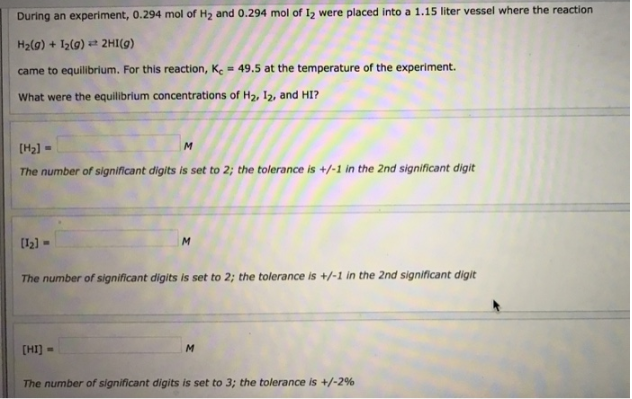# Problem: During an experiment, 0.294 mol of H2 and 0.294 mol of I2 were placed into a 1.15 liter vessel where the reaction H2(g) + I2(g) ⇌ 2HI(g) came to equilibrium. For this reaction, Kc = 49.5 at the temperature of the experiment. What were the equilibrium concentrations of H2, I2, and HI?

###### FREE Expert Solution
84% (7 ratings)###### Problem Details

During an experiment, 0.294 mol of H2 and 0.294 mol of I2 were placed into a 1.15 liter vessel where the reaction

H2(g) + I2(g) ⇌ 2HI(g)

came to equilibrium. For this reaction, Kc = 49.5 at the temperature of the experiment.

What were the equilibrium concentrations of H2, I2, and HI?